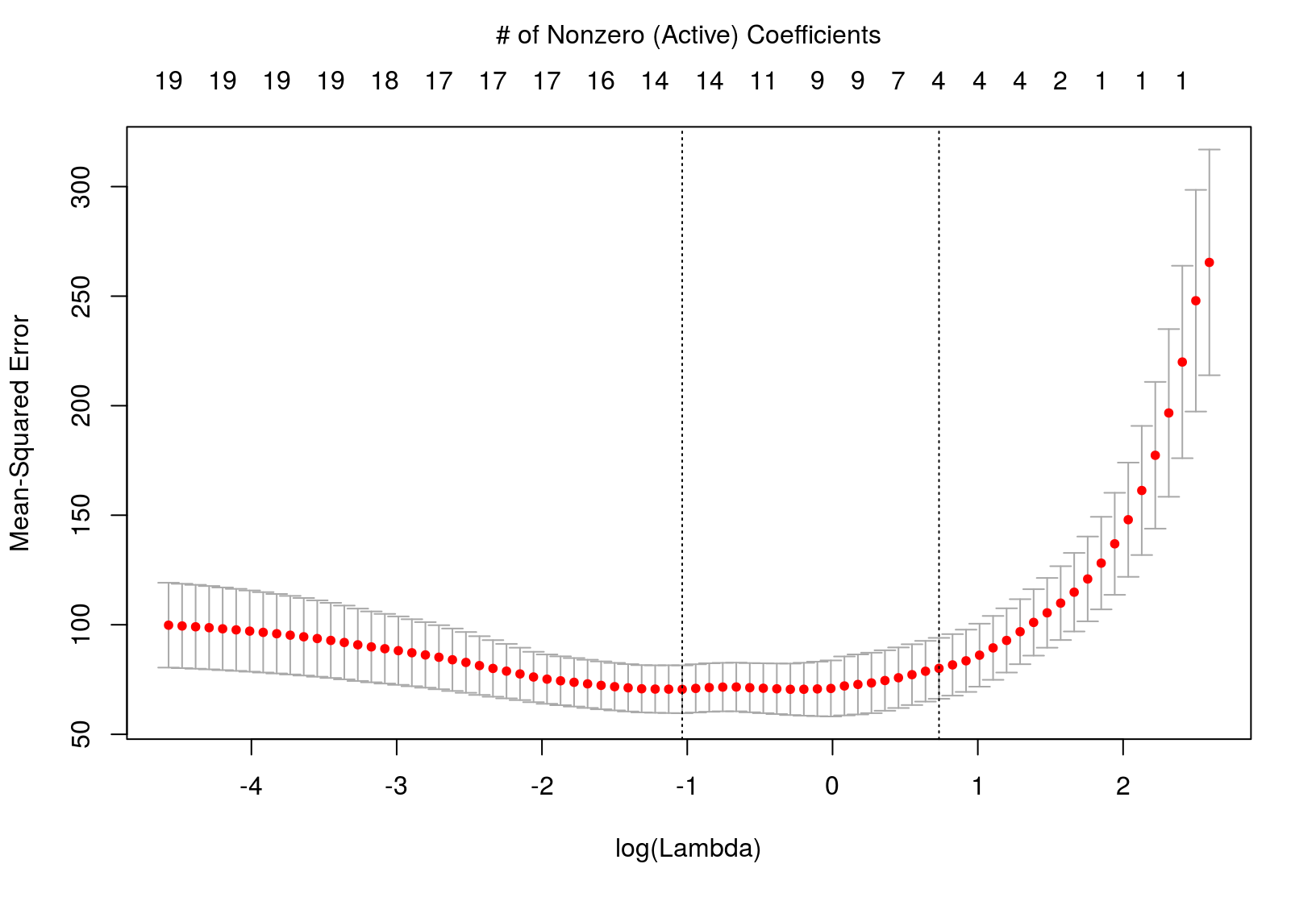September 19, 2014

$$\DeclareMathOperator*{\argmin}{arg\,min}$$

## Statistical Learning Group (SLG)

First of three statistical learning topics

• Today: Big picture talk
• Level of technicality will vary
• Sep. 26: Joshua Day - Comparison of Regularization Penalties
• Oct. 3: ?? - ??

• How can the SLG be improved?
• How can I be a more effective presenter?

## Outline

1. Review & Notation
2. Motivation
3. Regularized Regression
4. Implementation

## Supervised Learning

Recall from Justin's talk:

• Both inputs (features or predictors) & an output (response) are observed
• Want to build a learner (model) to understand the relationship between the two
• Often interested in prediction

## Notation

• $${y}_i$$: output or response variable
• $$i = 1,...,n$$
• $$x_{ij}$$: input, predictor, or feature variable
• $$j = 1,...,p$$

Matrix notation

$$\boldsymbol{y} = \begin{pmatrix} y_1 \\ y_2 \\ \vdots \\ y_n \end{pmatrix} \quad$$ and $$\quad \boldsymbol{X} = \begin{pmatrix} x_{11} & x_{12} & \cdots & x_{1p} \\ x_{21} & x_{22} & \cdots & x_{2p} \\ \vdots & \vdots & \cdots & \vdots \\ x_{n1} & x_{n2} & \cdots & x_{np} \end{pmatrix}$$

## Predictor Standardization

For regularized regression, predictors should be standardized before estimation

• Done using the classic "z-score" formula
• Puts each predictor on the same scale
• Mean of 0 and variance of 1
• Importance will be more clear later
• Use $$\hat{\beta_0} = \bar{y}$$ and estimate other coefficients
• Can transform back to original scale after estimation

## Estimation Goals

Given $$\boldsymbol{X}$$, find a function, $$f(\boldsymbol{X})$$, to model or predict $$Y$$

• Loss function, $$L(y, f(\boldsymbol{X}))$$
• Squared error loss is most common

$L(y, f(\boldsymbol{X})) = (y - f(\boldsymbol{X}))^2$

• Choose $$f$$ by minimizing the expected loss $E[(Y - f(\boldsymbol{X}))^2]$
• $$\Rightarrow f = E[Y | \boldsymbol{X} = \boldsymbol{x}]$$

## Linear Regression

Assume $$E[Y | \boldsymbol{X} = \boldsymbol{x}]$$ is a linear function, $$\displaystyle \sum_{j=1}^{p} x_{ij} \beta_j = \boldsymbol{x}_i'\boldsymbol{\beta}$$

• $$Y_i = \beta_0 + x_{i1}\beta_1 + x_{i2}\beta_2 + \dots + x_{ip}\beta_p + \epsilon$$

• Estimate $$\boldsymbol{\beta}$$ using ordinary least squares (OLS) $\hat{\boldsymbol{\beta}} = \argmin_{\boldsymbol{\beta}} \sum_{i=1}^n (y_i - \sum_{j=1}^{p} x_{ij} \beta_j)^2 \\ = \argmin_{\boldsymbol{\beta}} \| \boldsymbol{y} - \boldsymbol{X}\boldsymbol{\beta} \|^2_2$ $\Rightarrow \hat{\boldsymbol{\beta}}^{OLS} = (\boldsymbol{X}'\boldsymbol{X})^{-1} \boldsymbol{X}'\boldsymbol{y} \Rightarrow \hat{f}(\boldsymbol{x}_i) = \boldsymbol{x}_i'\hat{\boldsymbol{\beta}}$

## Linear Regression Shortcomings

Despite its wide use and elegant theory, linear regession has its shortcomings

• Prediction accuracy
• Often can be improved upon
• Model interpretability
• Linear model does not automatically do variable selection

## Assessing Prediction Accuracy

Given a new input, $$\boldsymbol{x}_0$$, how do we assess our prediction $$\hat{f}(\boldsymbol{x}_0)$$?

• Expected Prediction Error (EPE)
• \begin{aligned} EPE(\boldsymbol{x}_0) &= E[(Y_0 - \hat{f}(\boldsymbol{x}_0))^2] \\ &= \text{Var}(\epsilon) + \text{Var}(\hat{f}(\boldsymbol{x}_0)) + \text{Bias}(\hat{f}(\boldsymbol{x}_0))^2 \\ &= \text{Var}(\epsilon) + MSE(\hat{f}(\boldsymbol{x}_0)) \end{aligned}
• $$\text{Var}(\epsilon)$$: irreducible error variance
• $$\text{Var}(\hat{f}(\boldsymbol{x}_0))$$: sample-to-sample variability of $$\hat{f}(\boldsymbol{x}_0)$$
• $$\text{Bias}(\hat{f}(\boldsymbol{x}_0))$$: average difference of $$\hat{f}(\boldsymbol{x}_0)$$ & $$f(\boldsymbol{x}_0)$$

## Estimating Prediction Error

Common approach to estmating prediction error

• Randomly split data into "training" and "test" sets
• Test set has $$m$$ observations
• Calculate $$\hat{f}$$ using training data
• Estimate prediction error using test set MSE $\widehat{MSE}(\hat{f}) = \frac{1}{m} \sum_{i=1}^{m} (y_i - \hat{f}(x_i))^2$

Why?

• Want our model/predictions to perform well with new/future data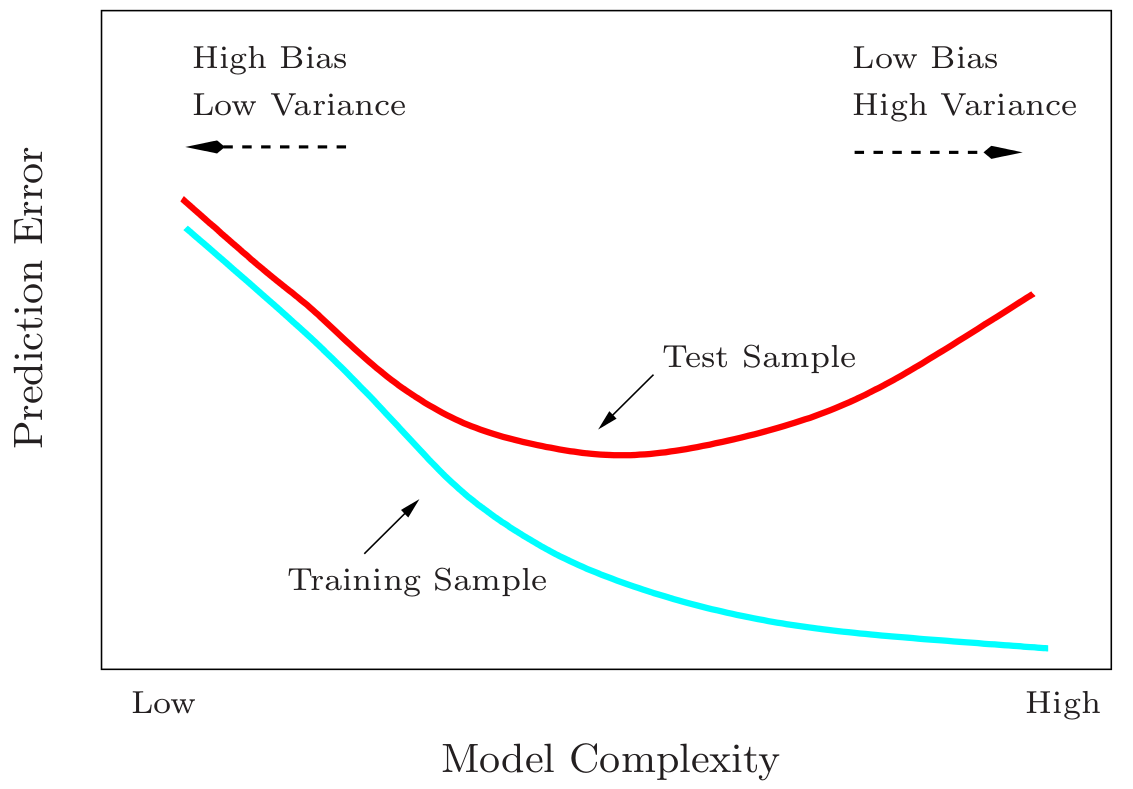Source: Hastie, Tibshirani, & Friedman (2009)

## Improving Prediction Accuracy

If $$f(x) \approx \text{linear}$$, $$\hat{f}$$ will have low bias but possibly high variance

• Correlated predictors
• $$p \approx n$$ or high-dimensional setting where $$p > n$$

Want to minimize $MSE(\hat{f}(x)) = \text{Var}(\hat{f}(x)) + \text{Bias}(\hat{f}(x))^2$

• Sacrifice bias to reduce variance
• Hopefully leads to decrease in $$MSE$$
• Regularization allows us to tune this trade-off

## Variable Selection

Often interested in using only a subset of the predictors

• Want to identify the most relevant predictors
• Usually the big picture is of interest
• Helps avoid an overly complex model that is difficult to interpret

Linear regression does not directly determine which predictors to use

How do we choose which predictors to use?

• Theory or expertise
• Ad hoc trial and error using p-values

## Automatic Subset Selection Methods

• Best subset
• Forward or backward stepwise
• Forward stagewise
• Pick the best subset using test set prediction $$MSE$$, $$C_p$$, AIC, or BIC

Still not ideal

• Discrete process: predictor is either included or excluded
• Unstable & highly variable
• Regularization is more continuous, & less variable as a result

## Regularization Framework

Same setup as before

• Given $$\boldsymbol{X}$$, find a function, $$f(\boldsymbol{X})$$, to model or predict $$y$$
• Assume linear model and use squared error loss

$\hat{\boldsymbol{\beta}}(\lambda) = \argmin_{\beta} \left\{\sum_{i=1}^n (y_i - \sum_{j=1}^{p} x_{ij} \beta_j)^2 + \lambda J(\boldsymbol{\beta})\right\}$

• $$\lambda \ge 0$$ is a regularization (tuning or penalty) parameter
• $$J(\boldsymbol{\beta})$$ is a user-defined penalty function
• Typically, the intercept is not penalized

## Role of the Penalty Term

Consider $$\displaystyle J(\boldsymbol{\beta}) = \sum_{j=1}^p \beta_j^2 = \| \boldsymbol{\beta} \|^2_2 \hspace{0.2cm}$$ (Ridge Regression)

Equivalent formulations $\hat{\boldsymbol{\beta}}(\lambda)^{RR} = \argmin_{\boldsymbol{\beta}} \left\{\sum_{i=1}^n (y_i - \sum_{j=1}^{p} x_{ij} \beta_j)^2 + \lambda \sum_{j=1}^p \beta_j^2 \right\}$

$\hat{\boldsymbol{\beta}}(t)^{RR} = \argmin_{\boldsymbol{\beta}} \sum_{i=1}^n (y_i - \sum_{j=1}^{p} x_{ij} \beta_j)^2 \\ \text{subject to} \sum_{j=1}^p \beta_j^2 \le t$

## Role of the Regularization Parameter

$$\lambda$$ directly controls the bias-variance trade-off:

• $$\lambda = 0$$ corresponds to OLS
• $$\lambda \rightarrow \infty$$ puts more weight on the penalty function and results in more shrinkage of the coefficients
• Introduce bias at the sake of reducing variance

Each $$\lambda$$ results in a different solution $$\hat{\boldsymbol{\beta}}(\lambda)$$

• Choosing $$\lambda$$ correctly is crucial (discussed later)

## The Lasso

Tibshirani (1996): Uses $$\displaystyle J(\boldsymbol{\beta}) = \sum_{j=1}^p |\beta_j| = \| \boldsymbol{\beta} \|_1$$

$\hat{\boldsymbol{\beta}}(\lambda)^{L} = \argmin_{\beta} \left\{\sum_{i=1}^n (y_i - \sum_{j=1}^{p} x_{ij} \beta_j)^2 + \lambda \sum_{j=1}^p |\beta_j| \right\}$

The subtle change in the penalty makes a big difference

• Not only shrinks coefficients towards zero, but sets some of them to be exactly zero
• Thus performs continuous variable selection
• Hence the name, Least Absolute Shrinkage and Selection Operator (LASSO)

## Geometric Interpretation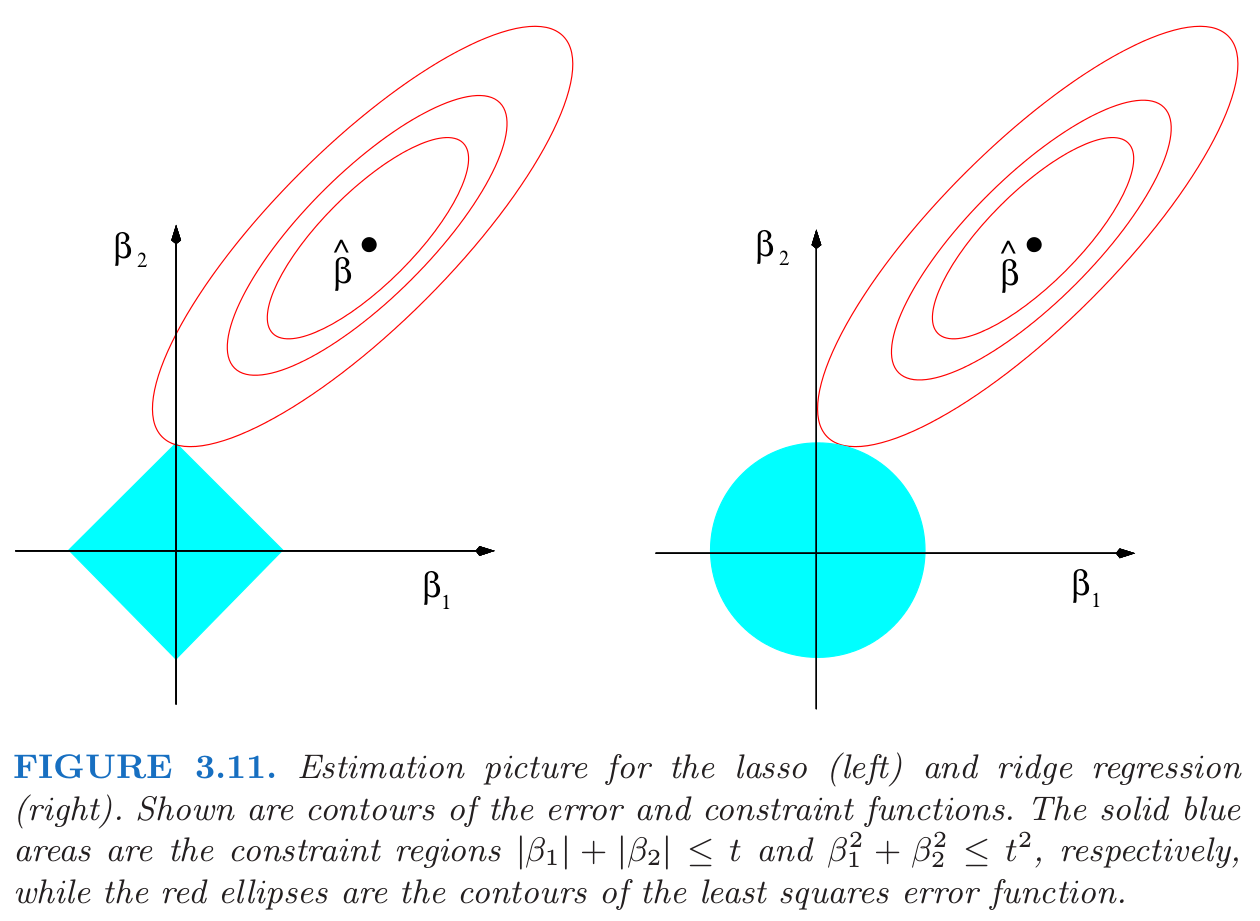Source: Hastie, Tibshirani, & Friedman (2009)

## General Regularization Framework

$\min_{f \in \mathcal{H}} \sum_{i=1}^n \left\{L(y_i, f(x_i)) + \lambda J(f)\right\}$

• $$\mathcal{H}$$ is a space of possible functions

Very general and flexible framework

• $$L$$: squared error, absolute error, zero-one, negative log-likelihood (GLM), hinge loss (support vector machines), …
• $$J$$: ridge regression, lasso, adaptive lasso, group lasso, fused lasso, thesholded lasso, generalized lasso, constrained lasso, elastic-net, dantzig selector, SCAD, smoothing splines, …
• Allows us to incorporate prior knowledge (sparsity, structure, etc.)

## Example: NCAA Dataset

• From Mangold, Bean, & Adams (2003) via Dr. Dennis Boos
• Contains information on 94 major NCAA division I universities
• $$y$$: average 6-year graduation rate for 1996-1998
• $$bbindex$$: author-created basketball index
• $$attend$$: average basketball attendance
• 17 other predictors suggested by the literature
• Acceptance rate, student-to-faculty ratio, etc.

Goal: Use OLS, ridge regression, and the lasso to find the best predictive model

Implementation: glmnet package in R

## Computational Considerations

Regularized regression estimates are a function of $$\lambda$$

Fortunately efficient algorithms exist

• Solution path algorithms
• LARS Algorithm for the Lasso (Efron et al., 2004)
• Piecewise linearity (Rosset & Zhu, 2007)
• Generaic path algorithm (Zhou & Wu, 2013)
• Others
• Pathwise coordinate descent (Friedman et al., 2007)
• Alternating Direction Method of Multipliers (ADMM) (Boyd et al. 2011)

## Lasso Solution Path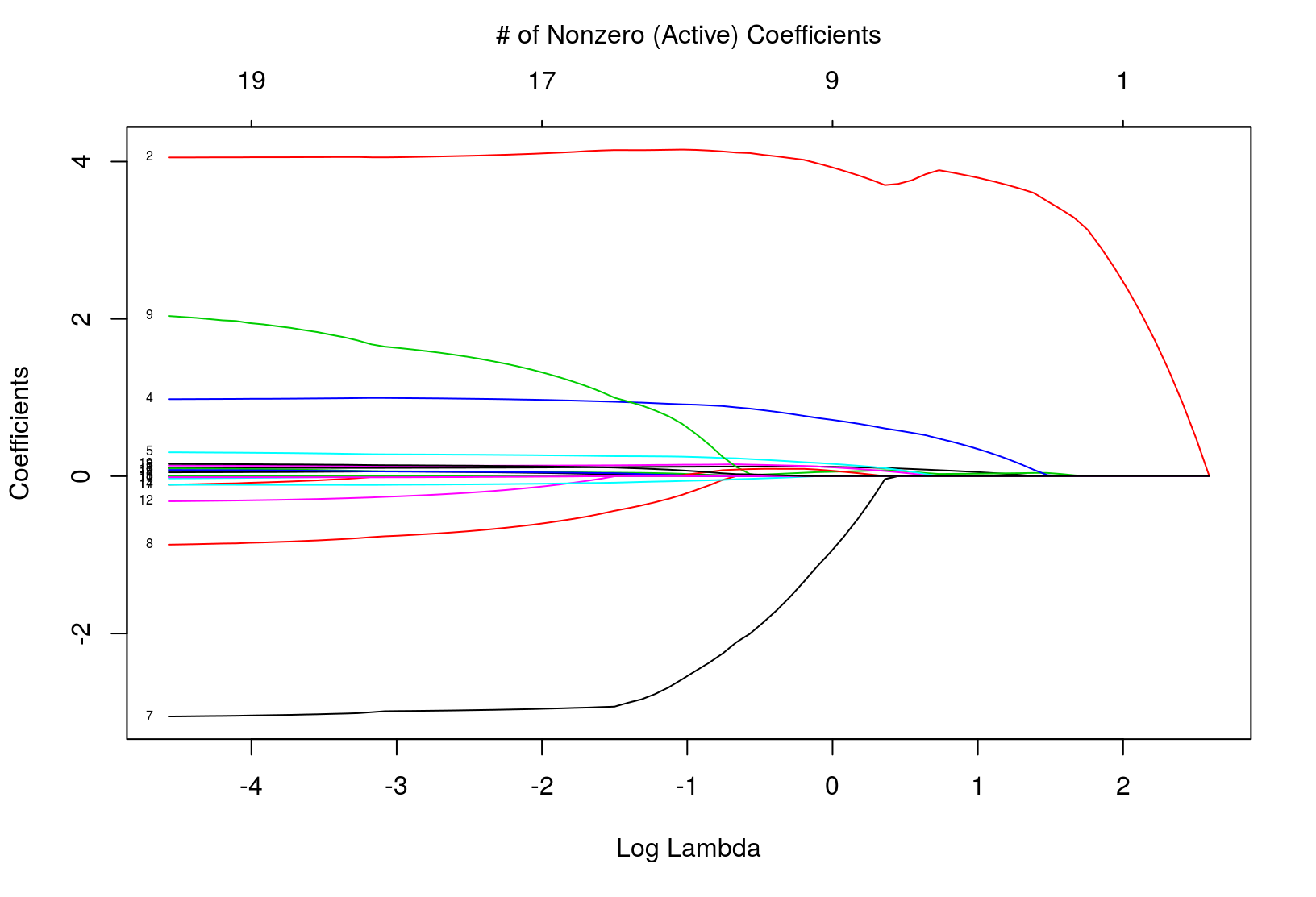## Ridge Regression Solution Path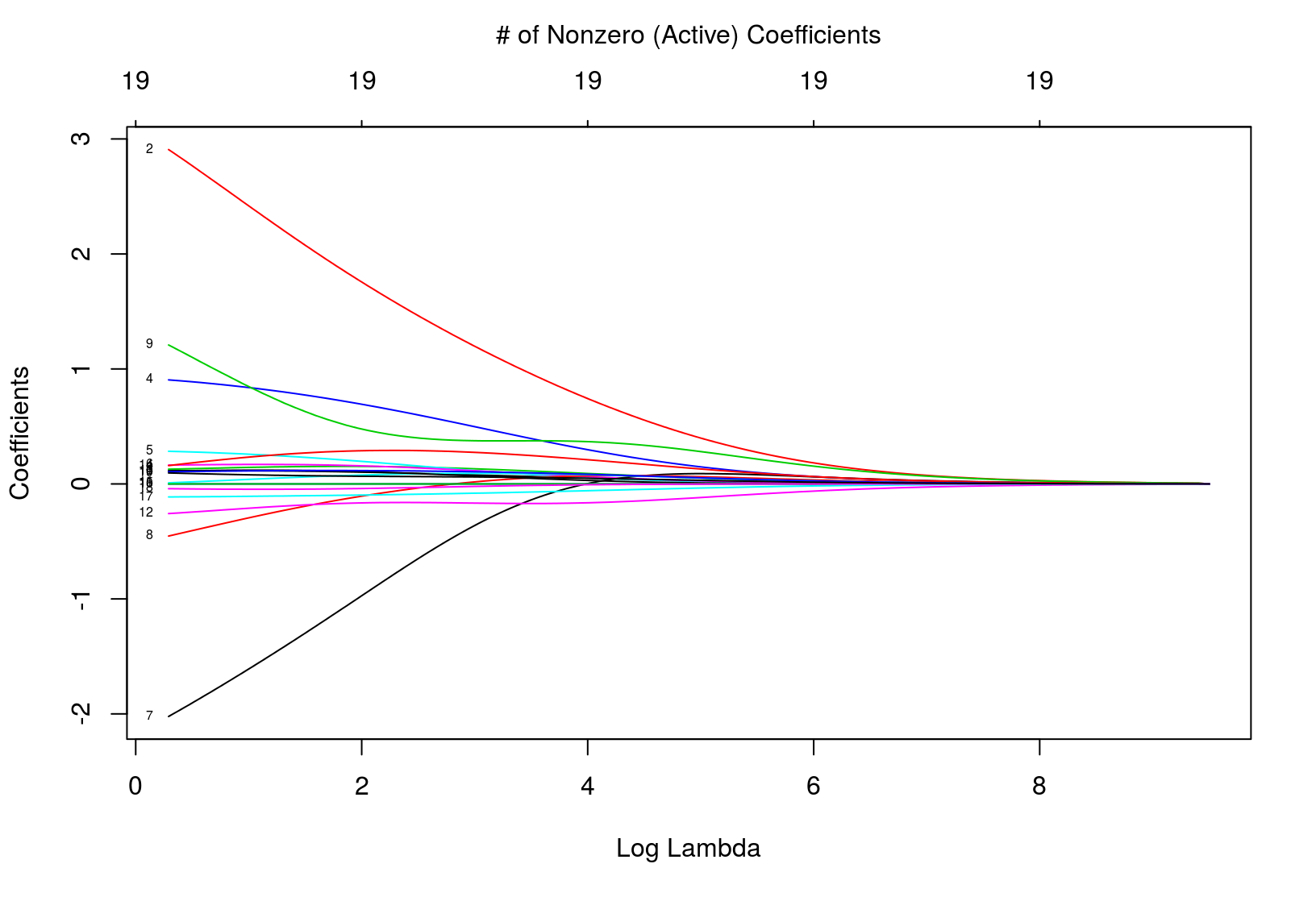## Choice of Regularization Parameter

Efficiently obtaining the entire solution path is nice, but we still have to choose $$\lambda$$

• Critical since $$\lambda$$ constrols the bias-variance tradeoff

• $$C_p$$, AIC, BIC, adjusted $$R^2$$

Cross validation is a popular alternative

• Choice based on predictive performance
• Makes fewer model assumptions
• More widely applicable

## Cross Validation Motivation

Ideally:• Separate validation set for choosing $$\lambda$$ for a given method
• Reusing training set encourages overfitting
• Using test set to pick $$\lambda$$ underestimates the true error
• Often we do not have enough data for a seperate validation set
• Cross validation remedies this problem

## $$K$$-Fold Cross Validation

1. Randomly split training data into $$K$$ parts ("folds")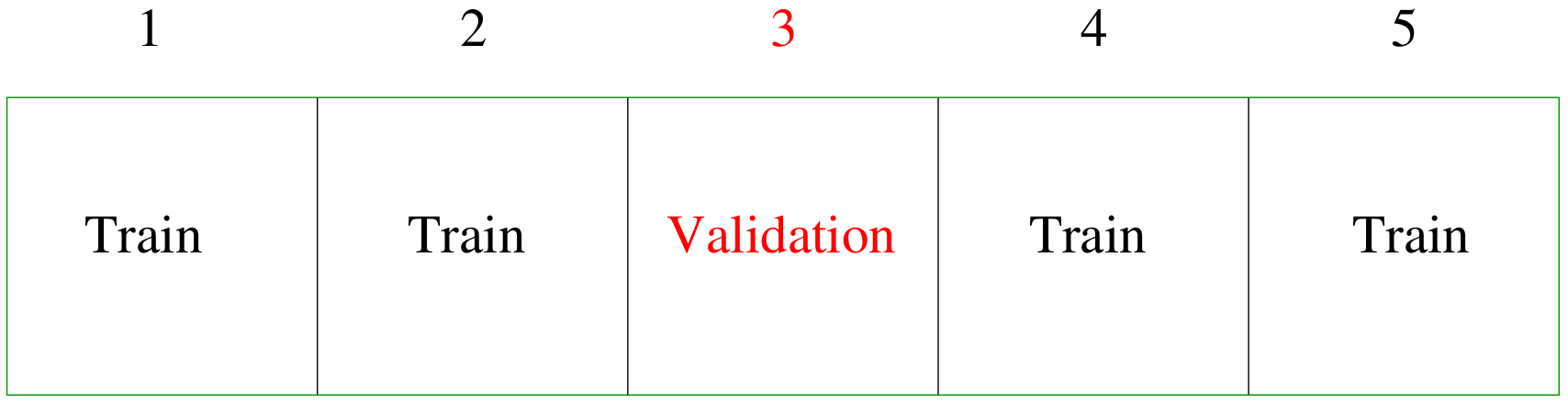2. Fit model using data in $$K-1$$ folds for multiple $$\lambda\text{s}$$
3. Calculate prediction MSE on remaining fold
4. Repeat process for each fold and average the MSEs
• Common choices of $$K$$: 5, 10, and $$n$$ (leave-one-out CV)
• One standard error rule
• Choose $$\lambda$$ corresponding to smallest model with MSE within one standard error of the minimum MSE

## Lasso 10-Fold Cross Validation